# SeriesBode

Series circuit with Bode analysis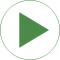# Information

This information is part of the Modelica Standard Library maintained by the Modelica Association.

The frequency of the voltage source is varied by a logarithmic ramp, the supply voltage magnitude is constant.

Plot versus `voltageSource.f` on a logarithmic scale in order to determine the Bode diagrams of the ratio of the voltage of the resistor divided by the supply voltage:

• Gain response: `dB_y`
• Phase response: `arg_y`

# Outputs (3)

abs_y Default Value: bode.abs_y Type: Real Description: Magnitude of voltage ratio Default Value: bode.dB_y Type: AmplitudeLevelDifference (dB) Description: Log10 of magnitude of voltage ratio in dB Default Value: bode.arg_y Type: Angle (rad) Description: Angle of voltage ratio

# Components (9)

frequencySweep voltageSource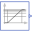Type: LogFrequencySweep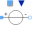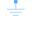Type: Ground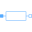Type: Resistor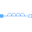Type: Inductor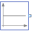Type: ComplexConstant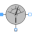Type: CurrentSensor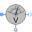Type: VoltageSensor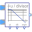Type: Bode